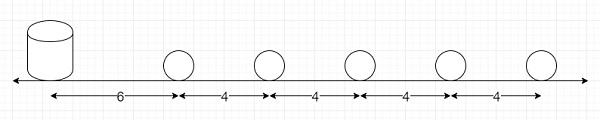# Find the distance covered to collect items at equal distances in Python

Suppose one race is going to be organized. Where different stones are placed on a road. One bucket is present at the starting point of the race, this is 6 units away from the first stone. The other stones are 4 units apart from each other and lie straight in a line one after another. Now, the participants start from the bucket, then collects the nearest stone, comes back and puts that stone into the bucket, after that runs again to collect the next nearest stone, runs back, and puts it in the bucket. This process will be continued until all the stones have been put into the bucket. If there are n stones, then we have to find total distance that need to be covered for the participants.So, if the input is like n = 5, then the output will be 140 as 2*6 + 2(6 + 4) + 2(6 + 4 + 4) + 2(6 + 4 + 4 + 4) + 2(6 + 4 + 4 + 4 + 4) = 140

To solve this, we have to solve this equation −

• For stone 1, we have to cover (6+6) = 2*6 distance

• For stone 2, we have to cover ((6+4)+(6+4)) = 2*(6+4) distance

• For stone 3, we have to cover ((6+4+4)+(6+4+4)) = 2*(6+4+4) distance

• For stone n, we have to cover ((6+4*(n-1))+(6+4*(n-1))) = 2*(6+4*(n-1)) distance

For all stones we have to cover −

• D = 2*6 + 2*(6+4) + 2*(6+4+4) + … + 2*(6+4*(n-1))

• D = 2*[6 + (6+4) + (6+2*4) + … + (6+(n-1)*4)]

• D = 2*[6n + 4(1 + 2 + … + (n-1))]

• D = 2*[6n + 4(n*(n-1)/2)]

• D = 2*[6n + 2(n*(n-1))]

## Example

Let us see the following implementation to get better understanding −

Live Demo

def find_distance(n):
return 2*(6*n + 2*((n-1)*n))
n = 5
print(find_distance(n))

## Input

5

## Output

140